# ethnobotanyR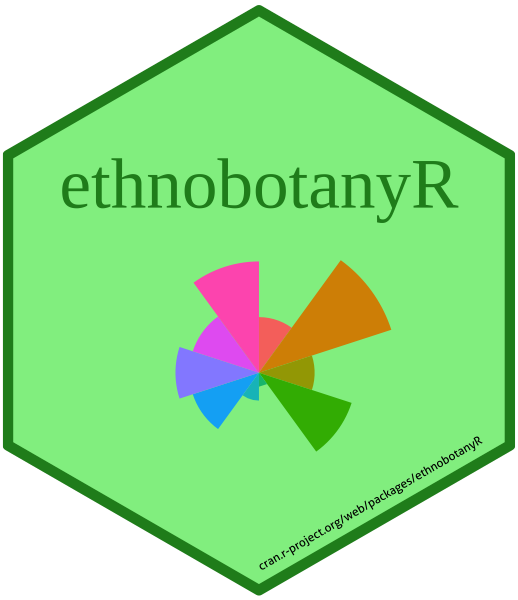The ethnobotanyR package can be used to calculate common quantitative ethnobotany indices to assess the cultural significance of plant species based on informant consensus. The package closely follows two papers, one by Tardio and Pardo-de-Santayana (2008) and another by Whitney et al. (2018).

An example data set called ethnobotanydata is provided to show what the layout for standard ethnobotany data should be like to interface with the ethnobotanyR package. This is an ethnobotany data set including one column of 20 knowledge holder identifiers informant and one of four species names sp_name. The rest of the columns are the identified ethnobotany use categories. The data in the use categories is populated with counts of uses per person (should be 0 or 1 values). 1

First six rows of the example ethnobotany data included with ethnobotanyR
informant sp_name Use_1 Use_2 Use_3 Use_4 Use_5 Use_6 Use_7 Use_8 Use_9 Use_10
inform_a sp_a 0 0 1 0 0 0 0 1 1 0
inform_a sp_b 0 0 0 0 0 0 0 0 0 0
inform_a sp_c 0 0 0 0 0 1 1 0 0 0
inform_a sp_d 0 0 0 0 0 0 0 0 0 0
inform_b sp_a 0 1 1 0 0 1 0 0 1 0
inform_b sp_b 1 0 0 0 0 0 1 0 0 0

## ethnobotanyR package functions

The use report URs() function calculates the use report (UR) for each species in the data set. $\begin{equation} UR_{s} = \sum_{u=u_1}^{^uNC} \sum_{i=i_1}^{^iN} UR_{ui} \end{equation}$

URs() calculates the total uses for the species by all informants (from $$i_1$$ to $$^iN$$) within each use-category for that species $$(s)$$. It is a count of the number of informants who mention each use-category $$NC$$ for the species and the sum of all uses in each use-category (from $$u_1$$ to $$^uNC$$).

ethnobotanyR::URs(ethnobotanydata)
#>   sp_name URs
#> 1    sp_c  52
#> 2    sp_a  43
#> 3    sp_d  43
#> 4    sp_b  36

Radial_plot() shows these results together with a radial plot for quick assessment of the result using the ggplot2 library.

ethnobotanyR::Radial_plot(ethnobotanydata, ethnobotanyR::URs)
#>   sp_name URs
#> 1    sp_c  52
#> 2    sp_a  43
#> 3    sp_d  43
#> 4    sp_b  36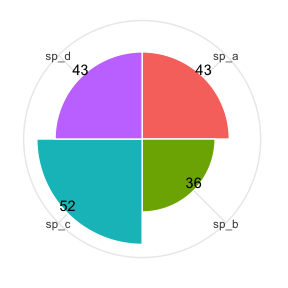The URsum() function calculates the sum of all ethnobotany use reports (UR) for all species in the data set.

ethnobotanyR::URsum(ethnobotanydata)
#>  "Sum of all Use Reports (UR) for all species in the data set"
#>  174
The CIs() function is run with calculates the Cultural Importance Index (CI) for each species in the data set. $\begin{equation} CI_{s} = \sum_{u=u_1}^{^uNC} \sum_{i=i_1}^{^iN} UR_{ui/N}. \end{equation}$

CIs() is essentially URs() divided by the number of informants to account for the diversity of uses for the species.

ethnobotanyR::Radial_plot(ethnobotanydata, ethnobotanyR::CIs)
#>   sp_name     CI
#> 1    sp_c 0.2364
#> 2    sp_a 0.1955
#> 3    sp_d 0.1955
#> 4    sp_b 0.1636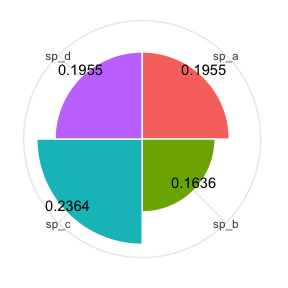The FCs() function calculates the frequency of citation (FC) for each species in the data set. $\begin{equation} FC_s = \sum_{i=i_1}^{^iN} UR_i \end{equation}$

FCs() is the sum of informants that cite a use for the species.

ethnobotanyR::Radial_plot(ethnobotanydata, ethnobotanyR::FCs)
#>   sp_name FCs
#> 1    sp_c  17
#> 2    sp_a  15
#> 3    sp_b  12
#> 4    sp_d  12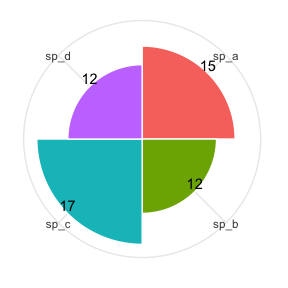The NUs() function calculates the number of uses (NU) for each species in the data set. $\begin{equation} NU_s = \sum_{u=u_1}^{^uNC} \end{equation}$

$$NC$$ are the number of use categories. NUs() is the sum of all categories for which a species is considered useful.

ethnobotanyR::Radial_plot(ethnobotanydata, ethnobotanyR::NUs)
#>   sp_name NUs
#> 1    sp_c   8
#> 2    sp_d   8
#> 3    sp_a   7
#> 4    sp_b   7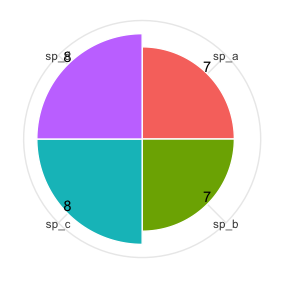The RFCs() function calculates the relative frequency of citation (RFC) for each species in the data set. $\begin{equation} RFC_s = \frac{FC_s}{N} = \frac{\sum_{i=i_1}^{^iN} UR_i}{N} \end{equation}$

$$FC_s$$ is the frequency of citation for each species $$s$$, $$UR_i$$ are the use reports for all informants $$i$$ and $$N$$ is the total number of informants interviewed in the survey.

ethnobotanyR::Radial_plot(ethnobotanydata, ethnobotanyR::RFCs)
#>   sp_name RFCs
#> 1    sp_c 0.85
#> 2    sp_a 0.75
#> 3    sp_b 0.60
#> 4    sp_d 0.60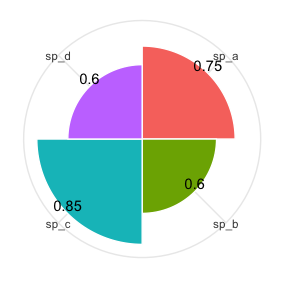The RIs() function calculates the relative importance index (RI) for each species in the data set. $\begin{equation} RI_s = \frac{RFC_{s(max)} + RNU_{s(max)}}{2} \end{equation}$

$$RFC_{s(max)}$$ is the relative frequency of citation for the species $$s$$ over the maximum, $$RNU_{s(max)}$$ is the relative number of uses for $$s$$ over the maximum.

ethnobotanyR::Radial_plot(ethnobotanydata, ethnobotanyR::RIs)
#>   sp_name   RIs
#> 1    sp_c 0.925
#> 2    sp_a 0.812
#> 3    sp_d 0.800
#> 4    sp_b 0.738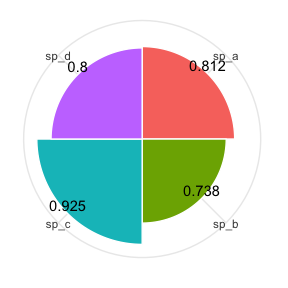The UVs() function calculates the use value (UV) index for each species in the data set. $\begin{equation} UV_{s} = \sum U_i/N \end{equation}$

$$U_i$$ is the number of different uses mentioned by each informant $$i$$ and $$N$$ is the total number of informants interviewed in the survey.

ethnobotanyR::Radial_plot(ethnobotanydata, ethnobotanyR::UVs)
#>   sp_name  UVs
#> 1    sp_c 2.60
#> 2    sp_a 2.15
#> 3    sp_d 2.15
#> 4    sp_b 1.80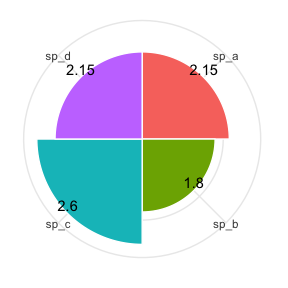The FLs() function calculates the fidelity level (FL) per species in the study. It is a way of calculating the percentage of informants who use a plant for the same purpose as compared to all uses of all plants.

$\begin{equation} FL_{s} = \frac {N_{s}}{UR_{s}} \end{equation}$

where $$N_s$$ is the number of informants that use a particular plant for a specific purpose, and $$UR_s$$ is the total number of use reports for the species (multiply FLs by 100 to get the percent FL, as it is reported in some studies).

ethnobotanyR::FLs(ethnobotanydata)
#>    sp_name variable       FLs
#> 1     sp_c    Use_8 23.076923
#> 2     sp_a    Use_3 20.930233
#> 3     sp_d    Use_8 20.930233
#> 4     sp_b    Use_7 19.444444
#> 5     sp_a    Use_2 18.604651
#> 6     sp_d    Use_7 18.604651
#> 7     sp_c    Use_1 17.307692
#> 8     sp_b    Use_1 16.666667
#> 9     sp_a    Use_6 16.279070
#> 10    sp_d    Use_3 16.279070
#> 11    sp_a    Use_8 13.953488
#> 12    sp_b    Use_3 13.888889
#> 13    sp_b    Use_6 13.888889
#> 14    sp_b    Use_9 13.888889
#> 15    sp_c    Use_2 13.461538
#> 16    sp_a    Use_5 11.627907
#> 17    sp_a    Use_9 11.627907
#> 18    sp_d    Use_1 11.627907
#> 19    sp_d    Use_6 11.627907
#> 20    sp_d    Use_9 11.627907
#> 21    sp_c    Use_4 11.538462
#> 22    sp_c   Use_10 11.538462
#> 23    sp_b    Use_4 11.111111
#> 24    sp_b    Use_5 11.111111
#> 25    sp_c    Use_7  9.615385
#> 26    sp_c    Use_6  7.692308
#> 27    sp_a   Use_10  6.976744
#> 28    sp_d    Use_2  6.976744
#> 29    sp_c    Use_5  5.769231
#> 30    sp_d    Use_5  2.325581

## ethnobotanyR chord diagrams with circlize

The following chord plots are made using functions from the circlize package. An example of the application of chord plots in ethnobotany is described by Whitney et al. (2018).

The ethnoChord() function creates a chord diagram of ethnobotany uses and species.

ethnobotanyR::ethnoChord(ethnobotanydata)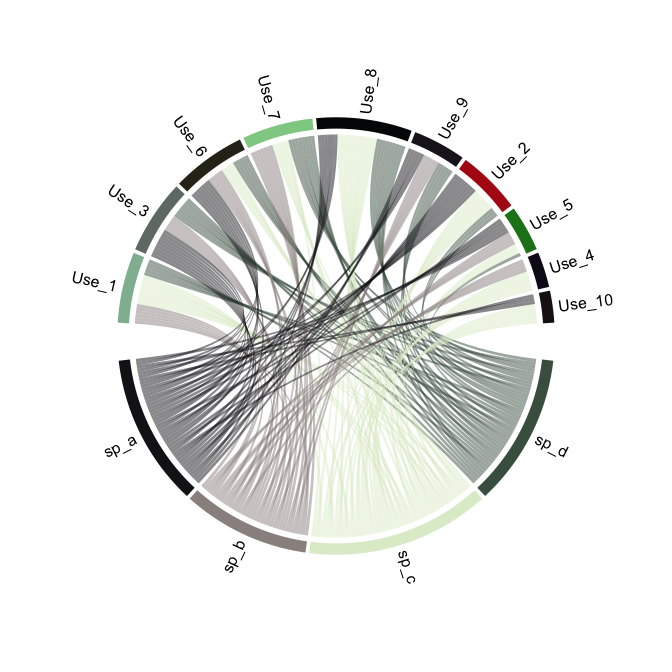#>  "Chord diagram for each use related to each species in the data set"

The ethnoChordUser() function creates a chord diagram of informants and species uses for ethnobotany studies.

ethnobotanyR::ethnoChordUser(ethnobotanydata)